# Numerical and Experimental Investigations on a Solar Chimney Integrated in a Building Facade

Numerical and Experimental Investigations on a Solar Chimney Integrated in a Building Facade

L. Cirillo D. Di Ronza V. Fardella O. Manca O. Manca

Dipartimento di Ingegneria Industriale e dell’Informazione, Seconda Università degli Sudi di Napoli, Via Roma 29, 81031 Aversa (CE)-ITALY

Corresponding Author Email:
sergio.nardini@unina.it
Page:
http://dx.doi.org/10.18280/ijht.330433
|
DOI:
246-254
|
Accepted:
|
Published:
31 December 2015
| Citation

OPEN ACCESS

Abstract:

In the paper, a numerical and experimental investigations on a prototypal solar chimney system integrated in a south facade of a building is presented. The chimney consists of a converging channel with one vertical wall and one inclined. The numerical analysis is carried out on a three-dimensional model in airflow and the governing equations are given in terms of k-ε turbulence model. The problem is solved by means of the commercial code Ansys-Fluent. The numerical analysis was intended to examine the effect of the solar chimney’s stack height and depth. Results are given in terms of wall temperature distributions, air velocity and temperature fields and transversal profiles for a uniform wall heat flux on the vertical wall. Thermal and fluid dynamics behaviors are evaluated in order to have some indications to improve the energy conversion system. Furthermore first results of an experimental investigation on a chimney system 4.0 m high and 1.5 m wide are given. Air velocity in the channel and surface temperature on the heated wall are presented.

(Presented at the AIGE Conference 2015)

Keywords:

Solar, CFD, Chimney, Nusselt number, Heat transfer.

1. Introduction

Solar chimney is a new method to produce electrical power and have been enhanced in recent two decades. It employs solar radiation to raise temperature of the air and the buoyancy of warm air to accelerate the air stream flowing through the system. By converting thermal energy into the kinetic energy of air movement, solar chimneys have a number of different applications such as ventilation, passive solar heating and cooling of buildings, solar-energy drying, and power generation. Moreover, it can be employed as an energy conversion system from solar to mechanical. A component, such as a turbine or piezoelectric component, set in the path of the air current, converts the kinetic energy of the flowing air into electricity.

Studies about thermal performance of solar chimney, are very important for engineering research. Schlaich  in the late 1970s was the first to propose the solar chimney concept. Less than 4 years after he presented his ideas at a conference and then construction of a pilot plant began in Manzanares, Spain, as a result of a joint venture between the German government and a Spanish utility. A 36 kW pilot plant was built, which produced electricity for 7 years, thus proving the efficiency and reliability of this novel technology. The chimney tower was 194.6 m high, and the collector had a radius of 122 m. Haaf  and Schlaich et al.  reported fundamental investigations, design criteria, and cost analysis for the Spanish system.

The phenomenon of secondary lows in a chimney caused by thermal instability has been much investigated, both numerically and experimentally [4-7]. Koonsrisuk and Chitsomboon  studied five mathematical models proposed in the literature with the numerical results of ANSYS-CFX and revealed that the roof diameter and chimney height are very important geometric dimensions for solar chimney design. Ming et al.  carried out steady axisymmetric numerical simulations on the solar chimney and found that the optimum height-to-diameter ratio (hc/dc) of a chimney ranges from 6 to 8. Kashiwa et al.  revealed that if hc/dc is not large enough, then the outside wind at the chimney top level have a strong influence on the air updraft inside the chimney. Pasumarthi and Sherif [10, 11] revealed that solar chimney technology is an alternative technology adapted to hot climate areas such as of Florida. They developed a mathematical model to estimate the temperature and power generation of solar chimney power plants. They show that greater power production is feasible by optimizing the collector roof shape and height. For unloaded condition which is the most common case investigated, the researchers [12-15] developed analytical models to estimate the performance of an SCPP with the assumption of a constant air density in the chimney. Arce et al.  carried out an experimental and numerical analysis on a small solar chimney.

Asnaghi and Ladjevardi  proposed a solar chimney in central region of Iran. High direct solar radiation in this region is available to develop a solar chimney to produce thermal and electrical energy. Dos Santos Bernardes et al  have presented a theoretical analysis of a solar radiat air heater to predict the thermo-hydraulic behavior of the device. This methodology allows a detailed visualization of the effects of geometric of optimal geometric and operational characteristics for such devices. Tan and Wong  studied the effect of the solar chimney’s stack height, width and inlet position on the interior performance. Pretorius and Kroger  have evaluated the influence of convective heat transfer equation, more accurate turbine inlet loss coefficient, quality collector roof glass and various types of soil on the performance of a large scale solar chimney power plant. Tahar and Mahfoud  carried out a numerical study of natural convection in a solar chimney. They have examined the effect of the system geometry on the natural convection phenomenon in the solar chimney. Zhou et al.  have built a pilot experimental solar chimney power with an air collector 10 m in diameter and an 8 m tall. Koonsrisuk in another work  studied sloped solar chimney system. The sloped collector was like a chimney, then the chimney height can be reduced and the construction cost would be reduced. Weinrebe et al.  have developed an analysis for the solar chimneys with analytical and numerical model, which described the performance of solar chimneys. The model developed to estimate power output of solar chimneys and the effect of ambient conditions and structural dimensions on the power output. Hami et al.  carried out a numerical analysis for air flow simulation in the solar chimney. Ong  have developed a mathematical model of a solar chimney. The physical model is similar to the Trombe wall. Guo et al.  have proposed a three-dimensional numerical approach incorporating the radiation solar load and turbine models to investigate their effects on system performance.

Sangi et al  have conducted a more detailed numerical analysis of a solar chimney power plant. Numerical simulations were performed for the geometry of the prototype in Manzanares. Patel et al.  carried out a numerical analysis on solar chimney to optimize the geometry of the major components of a SCPP. The collectors were tested with chimneys of different divergence angles and also different chimney inlet openings. The results show that the best configuration have achieved using the chimney with a divergence angle of 2°. Petela  have studied asimplified model of solar chimney power plant. He have studied responsive trends to the varying input parameters. Buonomo et al.  carried out a numerical investigation on a prototypal solar chimney system integrated in a south facade of a building. The chimney consisted of a converging channel with one vertical wall and one inclined of 2°. The analysis was carried out on a 3-D model in airflow and the governing equations are given in terms of k-ε turbulence model. Xu et al.  carried out a numerical investigation to study a solar chimney with 2 km radius and 1 km height. More recently Buonomo et al.  carried out a numerical investigation on a prototypal solar chimney system 50.0 m high integrated in a south facade of a building. The numerical analysis was intended to examine the effect of the glass wall inclination of the solar chimney. Thermal and fluid dynamics behaviors were evaluated in order to have some indications to improve the energy efficiency of the system.

In the present paper, a numerical and experimental investigations on a prototypal solar chimney system integrated in a south facade of a building is presented. The chimney consists of a converging channel with one vertical wall and one inclined. The numerical analysis is carried out on a three-dimensional model in airflow and the governing equations are given in terms of k-ε turbulence model. Results are given in terms of wall temperature distributions, air velocity and temperature fields and transversal profiles for a uniform wall heat flux on the vertical wall. Furthermore first results of an experimental investigation on a chimney system 4.0 m high and 1.5 m wide are given. Air velocity in the channel and surface temperature on the heated wall are presented.

2. Mathematical Description

The investigated configuration is reported in Fig.1. It consists of a channel constituted by a vertical wall of height L, on which is imposed a uniform heat flux, and by a glass wall, that is inclined to the vertical at an angle a. The inlet and the outlet section are bmax and bmin respectively, while the depth is equal to W. H is the distance of the inlet chimney section from the ground. Numerical values of the considered geometry are with reference to Fig. 1: L=4.0 m, H=1.0 m, W=1.5 m and bmin = 0.10 m, 0.20 m, 0.30 m and 0.40 m.

Steady, turbulent, three-dimensional natural convection flow are considered in a solar chimney. All thermo-physical fluid properties are assumed invariant except for the density in the buoyancy force term that can be adequately modeled by the Boussinesq approximation. The thermo-physical properties of the air are evaluated at the ambient temperature, T0, which is assumed to be 300 K in all cases. The ambient is assumed to be a black body at the temperature of 300 K. The compression work and viscous dissipation are assumed negligibly small. Thus, the governing equations can be written as:

$\bar{\nabla} \cdot \bar{V}=0$    (1)

$\rho \bar{V} \cdot \bar{\nabla} \bar{V}=\rho \bar{g}-\bar{\nabla} p+\bar{\nabla} \cdot \overline{\bar{\tau}}$    (2)

With $\overline{\bar{\tau}}$ the viscous stress tensor.

$\rho c_{p} \bar{V} \cdot \bar{\nabla} T=\lambda \nabla^{2} T$    (3)

In the present work the k-ε model proposed by Launder and Spalding  is considered. The turbulent dynamic viscosity

$\mu_{t}=\rho \cdot C_{\mu} \cdot f_{\mu} \cdot\left(\frac{k^{2}}{\varepsilon}\right)$     (4)

is calculated from the knowledge of the kinetic energy of turbulence, k, and turbulent kinetic energy dissipation rate, e, given from the following equations:

## 1.png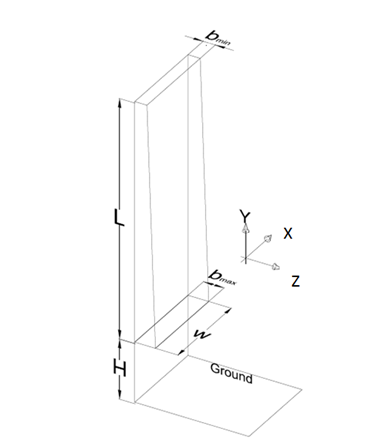Figure 1. Solar chimney scheme

Turbulence kinetic energy (k-equation)

$\frac{\partial}{\partial x}(\rho \bar{u} k)+\frac{\partial}{\partial y}(\rho \bar{v} k)+\frac{\partial}{\partial z}(\rho \bar{w} k)=\frac{\partial}{\partial x}\left[\left(\mu+\frac{\mu_{t}}{\sigma_{k}}\right) \frac{\partial k}{\partial x}\right]+\frac{\partial}{\partial y}\left[\left(\mu+\frac{\mu_{t}}{\sigma_{k}}\right) \frac{\partial k}{\partial y}\right]+\frac{\partial}{\partial z}\left[\left(\mu+\frac{\mu_{t}}{\sigma_{k}}\right) \frac{\partial k}{\partial z}\right]+G_{k}+G_{b}-\rho \varepsilon-D$      (5)

Turbulence dissipation (ε-equation)

$\frac{\partial}{\partial x}(\rho \bar{u} \varepsilon)+\frac{\partial}{\partial y}(\rho \bar{v} \varepsilon)+\frac{\partial}{\partial z}(\rho \bar{v} \varepsilon)=\frac{\partial}{\partial x}\left[\left(\mu+\frac{\mu_{t}}{\sigma_{s}}\right) \frac{\partial \varepsilon}{\partial x}\right]+\frac{\partial}{\partial y}\left[\left(\mu+\frac{\mu_{t}}{\sigma_{\varepsilon}}\right) \frac{\partial \varepsilon}{\partial y}\right]+\frac{\partial}{\partial z}\left[\left(\mu+\frac{\mu_{t}}{\sigma_{\varepsilon}}\right) \frac{\partial \varepsilon}{\partial z}\right]+C_{\varepsilon 1} f_{1} \frac{\varepsilon}{k}\left(G_{k}+G_{\varepsilon 3} G_{b}\right)-C_{\varepsilon 2} f_{2} \frac{\varepsilon^{2}}{k}+E$         (6)

The near-wall option employed in this investigation is the Standard Wall Functions proposed by Launder and Spalding .

3. Numerical Procedure

Ansys-Fluent  is selected as the computational software to solve the physical model as in [33, 36-38]. The computational domain has an external reservoir (at the inlet section) in order to simulate the diffusions of momentum and energy that occur outside the channel, as suggested by studies on vertical converging channels . Mainly were imposed conditions of no-slip and impermeability to the rigid walls, in addition to the specified wall heat flux. Ambient conditions were assumed at the inlet and outlet sections (ambient pressure and temperature). The operating temperature was set equal to 300 K; the acceleration of gravity was considered, and its value was set equal to 9.81 m/s2. All the non-heated surfaces were considered adiabatic whereas a uniform heat flux of 300 W/m2 on the heated surface was considered.

The second-order upwind scheme was chosen for unsteady energy and momentum equations. The Semi-Implicit Method for Pressure-Linked Equations (SIMPLE) scheme was chosen to couple pressure and velocity. Similar considerations were made for the choice of the discrete transfer radiation model (DTRM), assuming all surfaces to be diffuse [35, 40]. This means that the reflection of incident radiation at the surface is isotropic with respect to the solid angle. Therefore, the scattering effect is not taken into account. The equation for the exchange of radiation intensity of DTRM, dI, along path dscan be written as:

$\frac{\mathrm{d} I}{\mathrm{d} s}+\alpha I=\frac{\alpha \sigma \mathrm{T}^{4}}{\pi}$    (7)

Where: a is the absorption coefficient of the gas; I is the total radiation intensity that depends on the position (r) and the direction (s); T is the local temperature of the gas; σ is the Stefan-Boltzmann constant, 5.672·10-8 W/ (m2·K4). It is assumed that the refractive index is unitary.

The DTRM integrates the equation (6) along a set of rays that leave the outer walls. Mainly the number of rays traced and the computational grid limit the model’s accuracy. It was realized, however, an analysis in order to make a weighted choice of the number of divisions of the solid angle, as required by the chosen radiation model (DTRM). The goal was to achieve a compromise between the two requirements, reliability of the results and containment of calculation time.

Configurations with 33, 66 and 99 subdivisions of polar and azimuthal angles were considered to evaluate the discretization effect on the radiative heat transfer. Cases with 6 and 9 divisions gave almost the same results. Given the size of the file tracking, we opted for the model with 6 divisions which involves a greater speed in the reading of the same file and a reduction in computation time. Computation starts with zero values of the velocity components and with pressure and temperature values equal to the ambient ones. Convergence criteria of 10-6 and 10-8 for the residuals of the velocity components and energy were assumed. The sensitivity analysis of the grid was reported in Buonomo et al. .

4. Experimental Apparatus

The experimental apparatus was built in order to allow a considerable flexibility. The distance “I” shown in Fig. 2 can be adjusted from 10 cm until to 65 cm. The tilted glass wall can be inclined of an angle α, up to be in contact with the heated wall. The uniform distribution of the temperature has been obtained by means of two independent systems: 1) a condensing GPL boiler with a nominal power of 25 kW; 2) eight resistors of 220 Volt, with nominal power of 2 kW each. The fluid circulating in a number of tubes is water. The pumping power has been achieved by a centrifugal pump of 2 kW. The heat losses have been reduced by a panel of polyurethane foam, 7 cm thick located on the back of the system. It is possible to vary the geometry of the channel and the wall temperature, Fig.3. To regulate the temperature of the heat transfer fluid there is a boiler thermostat and a resistor thermostat. It is also possible to vary the water flow with a lamination valve. The hot fluid slide the wall from top to bottom so as to obtain the thermal uniformity. Figure 4 shows some pictures of the prototype of solar chimney designed e investigated in this work.

## 3.png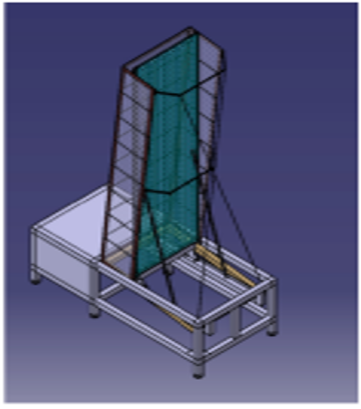Figure 3. 3D scheme of the experimental apparatus

## 4.png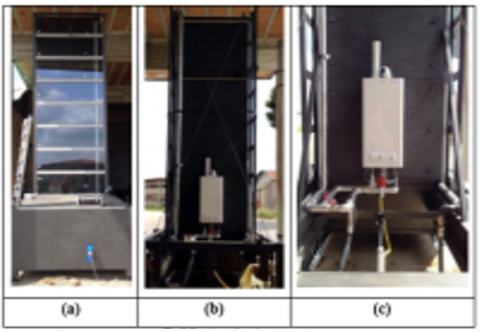Figure 4. Solar chimney prototype: a) front image of the channel; b) back image with condensing boiler; c) boiler and pipes details

4.1 Data acquisition

To control the parameters of interest, the plant has been equipped with appropriate tools; in particular, wall and ambient temperature were measured by means of type “J” thermocouples (Cu-Ni, sensitivity average of 52μV/°C, accuracy +/- 0.1°C). To determine that there wasa uniform wall temperature, nine thermocouples have been checked to the following order (in the middle of the heated wall): y=0, 25, 50, 100, 150, 200, 250, 300, 350 cm.

The ambient temperature has been measured near the test section, in an unperturbed zone. To evaluate the velocity in the channel, an anemometer Delta Ohm - HD 29V371TC25 has been used. The probe has a temperature and relative humidity sensor. The accuracy is +/- 2% of full scale (in the range 0m/s – 2m/s). The sensor has been placed in the channel 50 cm from the top edge. Agilent 34972A is the acquisition system.

## 2.png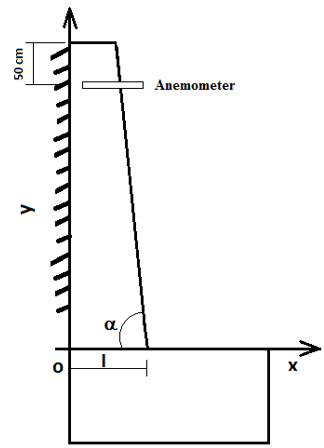Figure 2. Simplified scheme of the solar chimney

5. Results

5.1 Numerical results

The analysis was carried out by varying the thickness of the outlet section and keeping constant the inclination of the transparent wall, equal to 2° to the vertical. Figure 5 shows the temperature fields of the heated wall for different spacing of the chimney. The wall temperature increases from the bottom upwards due to the progressive heating of the air in the channel. Furthermore, it can be seen also that the temperature assumes a uniform value downstream the middle of channel height. We can observe how the wall temperatures decrease with increasing spacing of the channel.

## 5.png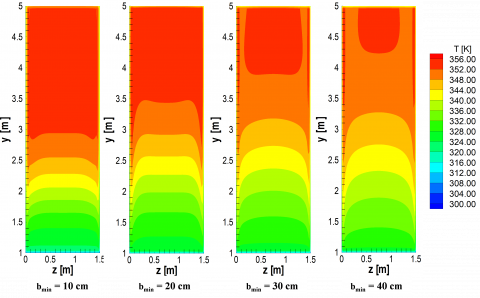Figure 5. Temperature fields of the heated wall for different chimney spacing

The differences are fairly small, as illustrated by the comparison between temperature trends along the channel on the centerline of the heated wall in Fig. 6. Temperature increases with the height except near the outlet section for the effect of the edge. Velocity profiles at outlet section of the channel is presented in Fig. 7. Velocities have very similar trends for different configurations. Decreasing the spacing of the channel, however, an increase in velocity. Table 1 compares main thermo-fluid dynamics parameters for the investigated configurations.

## 6.png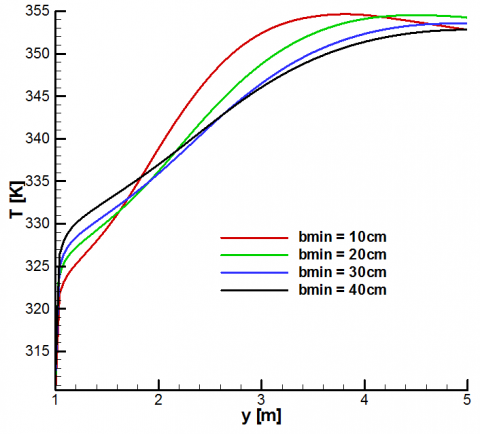Figure 6. Temperature profiles along the centerline of the heated wall for different channel spacings

## 7.png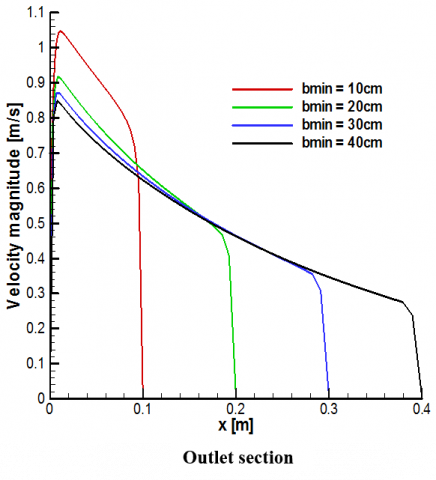Figure 7. Vertical velocity component profile at the centerline of the exit channel section for different channel spacings

Table 1. Thermo-fluid dynamics parameters

 bmin [m] 0.1 0.2 0.3 0.4 Heatflux [W/m2] 300 300 300 300 Average Nusselt number 26.6 53.6 81 106.5 Mass flow rate [g/s] 155 227 282 320 vmax[m/s] 1.05 1.012 0.964 0.978 Tsmax [K] 355 356 354 354

5.2 Experimental results

Experimental investigations have been performed in order to validate the numerical model. Results are presented in terms of surface temperature and air velocity in function of time. The investigated configurations are reported in Table 2, where the surface values at the inlet and outlet section are also reported. The configurations differ for the value of maximum channel gap attained in the inlet section whereas the inclination angle of the glass plate is 2° in all configuration. The increment of the temperature of the heated wall respect the ambient temperature is 40°C, value equal to the average temperature in the numerical investigation.

Configuration 1

Fig. 8 shows the story of surface temperatures of the heated wall, maximum air velocity in the section at 50 cm from the outlet section and average air velocity adjacent the heated wall at the channel exit. Figure 8 shows that the surface temperature of the heated wall becomes constant after 70 minutes. Since the motion is turbulent velocity presents irregular fluctuations as shown in Fig. 8a. However, the average air velocity increases with temperature. In the Fig. 8b is evident this behavior of the air velocity. The air velocity values have been averaged in function of the time each 65 min and becomes constant at nearly the same time at which wall temperature becomes constant.

Fig. 9 shows the history of the temperature of heated wall and air velocity adjacent the heated wall with a time step of 0.5 s. The oscillations of temperature and velocity values due to turbulence are clear. The right line is the average temperature and velocity. Fig. 9a shows that the values of temperatures are stable, while Fig. 9b shows the value of the air velocity fluctuates around the value of 0.96 m/s. At the output section the maximum value is 1.1 m/s.

Configuration 2

Similarly to previous configuration, Fig. 10 shows the average temperatures profile of the wall and the air velocity in the channel, as a function of time for Configuration 2 with bmax=0.34m. It worth noticing that this configuration is characterized by a value of 0.20 m of bmin equal to that of the numerical investigation.

Table 2. Investigated configurations

 bmax (m) Tw-Tamb(°C) α (°) Sin (m2) Sout (m2) 1 0.24 40 2 0.36 0.15 2 0.34 40 2 0.51 0.30 3 0.44 40 2 0.66 0.45 4 0.54 40 2 0.81 0.60

## 8a.jpg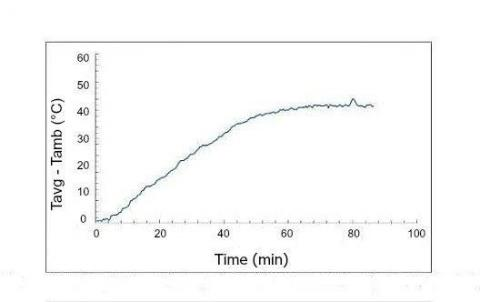(a)

## 8b.jpg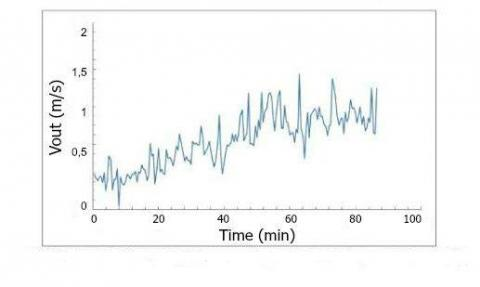(b)

Figure 8. Measured variables, time step of 30 s, as a function of time, for bmax=0.24 m: (a) heated wall temperature, (b) maximum velocity in the section at 50 cm from the outlet section

## 9a.jpg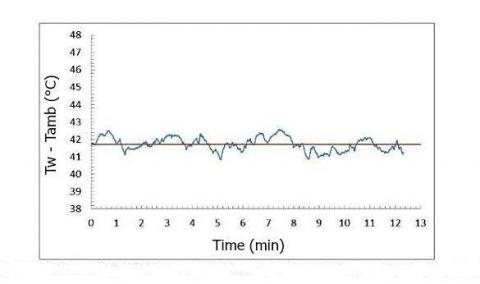(a)

## 9b.jpg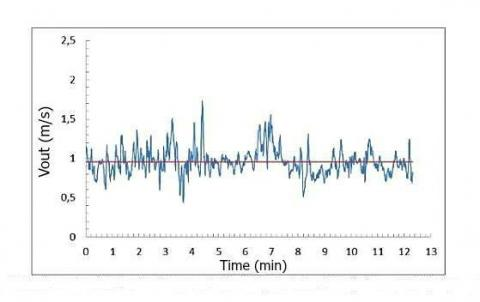(b)

Figure 9. Measured variables, time step of 0.5 s, as a function of time, for bmax=0.24 m: (a) heated wall temperature, (b) maximum velocity in the section at 50 cm from the outlet section

## 10a.jpg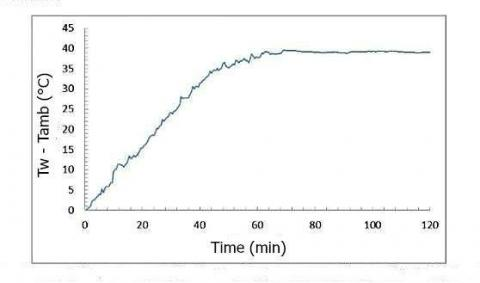(a)

## 10b.jpg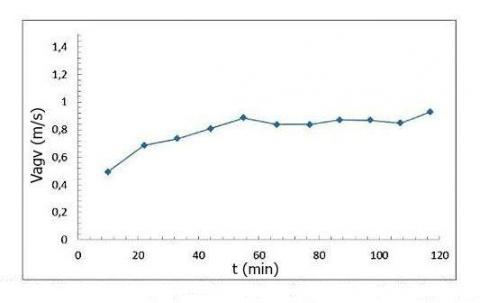(b)

Figure 10. Measured variables, time step of 30 s, as a function of time, for bmax=0.34 m: (a) heated wall temperature, (b) maximum average velocity in the section at 50 cm from the outlet section

Also in this configuration the asymptotic values of average wall temperature and velocity are attained after about 70 min. Fig. 11 shows the history of temperature and velocity with a time step of 0.50 s. The value of the air velocity fluctuates around the value of 0.92 m/s. At the output section the maximum value is 0.98 m/s that is very similar to the ne obtained from the numerical investigation.

Configuration 3-Fig. 12 shows the history of temperature and velocity with a time of 0.30 seconds for the third configuration (bmax=0.44m). The value of the air velocity fluctuates around the value of 0.85 m/s. At the output section the maximum velocity value is 0.93 m/s.

Configuration 4- Fig. 13 presents the trend of temperature and velocity with a time of 0.30 seconds for the fourth configuration (bmax=0.54m). The value of the air velocity fluctuates around the value of 0.77 m/s. At the output section the maximum velocity value is 0.80 m/s.

Finally, the average maximum air velocity valuesin the section at 50 cm from the outlet os a function of the maximum channel gap is reported in Fig. 14. As the channel increases the values of maximum air velocity decreases because the channel volume increase.

6. Conclusions

A numerical and experimental investigation on natural convection in air in a convergent chimney was carried out. Results of numerical investigation are given in terms of wall temperature distributions and air velocity at the exit section for an uniform wall heat flux on the vertical wall whereas the experiments were carried out for a uniform  temperature of the heated  wall and  for different channel  spacing. Results of numerical investigation are in good agreement with results from experiments. Analysis of the results showed that the characteristic thermo-fluid dynamic parameters of the phenomenon are strictly dependent on the chimney geometry. The solar chimney spacing have a considerable influence on the air speed inside the duct. Reducing channel spacing a velocity increase is obtained. If the aim of the solar chimney is the conversion from solar to mechanical energy higher velocity values are worthwhile so lower values of channel gap are better.

## 11a.jpg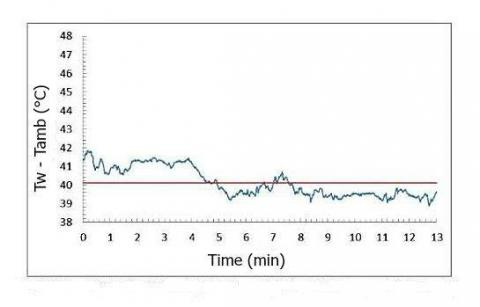(a)

## 11b.jpg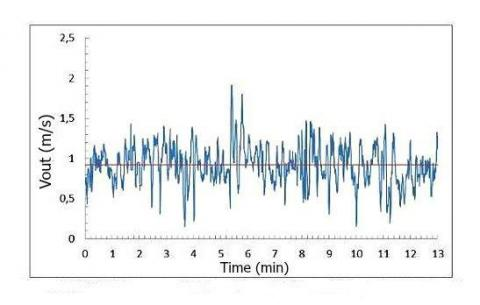(b)

Figure 11. Measured variables, time step of 0.5 s, as a function of time, for bmax=0.34 m: (a) heated wall temperature, (b) maximum velocity in the section at 50 cm from the outlet section

## 12a.jpg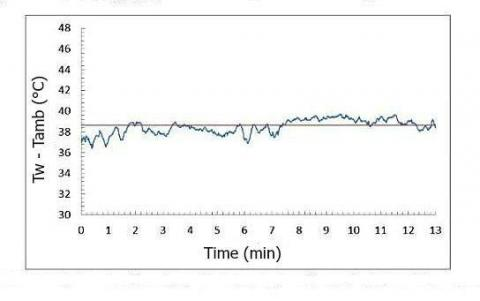(a)

## 12b.jpg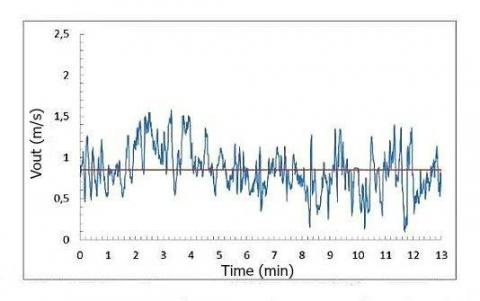(b)

Figure 12. Measured variables, time step of 0.5 s, as a function of time, for bmax=0.44 m: (a) heated wall temperature, (b) maximum velocity in the section at 50 cm from the outlet section

## 13a.jpg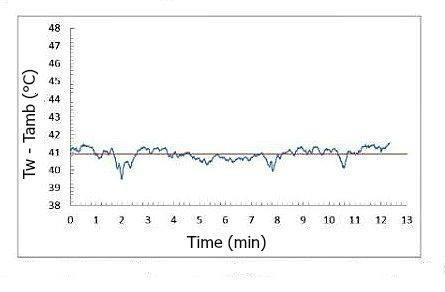(a)

## 13b.jpg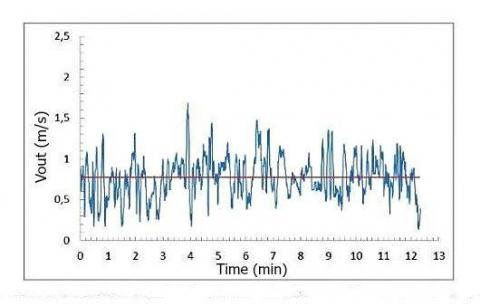(b)

Figure 13. Measured variables, time step of 0.5 s, as a function of time, for bmax=0.54 m: (a) heated wall temperature, (b) maximum velocity in the section at 50 cm from the outlet section

## 14a.jpgbmax (cm)

Figure 14. Average of maximum velocity in the section at 50 cm from the outlet section as a function of bmax

Acknowledgement

This study was supported by the Ministerodell’Ambiente e dellaTutela delTerritorio e del Mare with the 2010 grant research program S.E.E.M. (Solar EcoefficientEnvelope Model).

Nomenclature
 A, B, C coefficients b        [m] thickness H       [m] distance from ground L        [m] length of channel n normal direction S        [m2] surface T        [°C] temperature v        [m/s] air velocity x   [m] distance from the heated wall w  [m] width Greek symbol α        [°] angle of inclination ∂ partial differential φ scalar ρ        [kg/m3] density Г        [m2/s] diffusivity Subscripts w wall amb ambient in inlet out outlet max maximum value
References

1. J. Schlaich, The Solar Chimney: Electricity from the Sun, Edition Axel Menges, Stuttgart, Germany, 1995.

2. W. Haaf, K. Friedrich, G. Mayer, J. Schlaich, “Solar chimneys Part I: principle and construction of the pilot plant in Manzanares,” Int. J. Solar Energy, vol. 2, pp. 3-20, 1983. DOI: 10.1080/01425918308909911.

3. J. Schlaich, R. Bergermann, W. Schiel and G. Weinrebe, “Design of commercial solar updraft tower systems e utilization of solar induced convective flows for power generation,” ASME J. Solar Energy Eng., vol. 127, pp.117-24, 2005. DOI: 10.1115/1.1823493.

4. A.J. Gannon and T.W. von Backström, “Solar chimney cycle analysis with system loss and solar collector performance,” ASME J. Solar Energy Eng., vol. 122, pp. 133–7. DOI: 10.1115/1.1314379, 2000.

5. H. Pastohr, O. Kornadt and K. Gurlebeck, “Numerical and analytical calculations of the temperature and flow field in the upwind power plant,” Int. J. Energy Res. vol. 28, pp. 495–510, 2004. DOI: 10.1002/er.978.

6. T. Chitsomboon, “The effect of chimney-top convergence on efficiency of a solar chimney,” Proc. 13th Nat. Mech. Eng. Conf., Thailand, 1999.

7. A. Koonsrisuk and T. Chitsomboon, “Accuracy of theoretical models in the prediction of solar chimney performance,” Solar Energy, vol. 83, pp. 764-71. DOI: 10.1016/j.solener.2009.05.012, 2009.

8. T. Ming, R.K. de Richter, F. Meng, Y. Pan and W. Liu, “Chimney shape numerical study for solar chimney power generating systems,” Int. J. Energy Res., vol. 37, pp. 310-322, 2013. DOI: 10.1002/er.1910.

9. B.A. Kashiwa and C.B. Kashiwa, “The solar cyclone: a solar chimney for harvesting atmospheric water,” Energy, vol. 33, pp. 331-339, 2008. DOI: 10.1016/j.energy.2007.06.003.

10. N. Pasumarthi and S.A. Sherif, “Experimental and theoretical performance of a demonstration solar chimney model—Part I. Mathematical model development,” Int. J. Energy Res., vol. 22, pp. 277–288, 1998.

11. N. Pasumarthi and S.A. Sherif, “Experimental and theoretical performance of a demonstration solar chimney model-Part II. Experimental and theoretical results and economic analysis,” Int. J. Energy Res., vol. 22, pp. 443–461, 1998.

12. T.Z. Ming, W. Liu and G.L. Xu, “Analytical and numerical investigation of the solar chimney power plant systems,” Int. J. Energy Res., vol. 30, pp. 861-873, 2006. DOI: 10.1002/er.1191.

13. J.K. Yang, X.P. Zhou, B. Xiao and G.X. Hou, “Simulation of a pilot solar chimney thermal power generating equipment,” Renew. Energy, vol. 32, pp. 1637-1644, 2007. DOI: 10.1016/j.renene.2006.07.008.

14. Y.J. Dai, H.B. Huang and R.Z. Wang, “Case study of solar chimney power plants in northwestern regions of China,” Renew. Energy, vol. 28, pp. 1295-1304, 2003. DOI: 10.1016/S0960-1481(02)00227-6.

15. J. Arce, J.P. Xamán, G. Alvarez, M.J. Jiménez, R. Enríquez and M.R. Heras, “A simulation of the thermal performance of a small solar chimney already installed in a building,” Proc. ASME 4th Int. Conf. on Energy Sustainability, vol. 2, pp. 337-347, 2010. DOI: 10.1115/ES2010-90271.

16. A. Asnaghi and S.M. Ladjevardi, “Solar chimney power plant performance in Iran,” Renew Sust. Energy Rev., vol. 16, pp. 3383-3390, 2012. DOI: 10.1016/j.rser.2012.02.017.

17. M.A. Dos Santos Bernardes, R.M. Valle and M.F. Cortez, “Numerical analysis of natural laminar convection in a radial solar heater,” Int. J. Thermal Sciences, vol. 38, pp. 42-50, 1999. DOI: 10.1016/S0035-3159(99)80015-4.

18. A.Y.K. Tan and N.H. Wong, “Parameterization studies of solar chimneys in the tropics,” Energies, vol. 6, pp. 145-163, 2013. DOI: 10.3390/en6010145.

19. J.P. Pretorius and D.G. Kroger, “Critical evaluation of solar chimney power plant performance,” Solar Energy, vol. 80, pp. 535-544, 2006. DOI: 10.1016/j.solener.2005.04.001.

20. T. Tahar and D. Mahfoud, “Numerical simulation of natural convection in a solar chimney,” Int. J. Renew. Energy Res., vol. 2, pp. 712-717, 2012.

21. X. Zhou, J. Yang, B. Xiao and G. Hou, “Experimental study of temperature field in a solar chimney power setup,” Appl. Thermal Eng., vol. 27, pp. 2044-2050, 2007. DOI: 10.1016/j.applthermaleng.2006.12.007.

22. A. Koonsrisuk, “Mathematical modeling of sloped solar chimney power plants,” Energy, vol. 47, pp. 582-589, 2012. DOI: 10.1016/j.energy.2012.09.039.

23. M.A. Dos Santos Bernardes, A. Voss and G. Weinrebe, “Thermal and technical analyses of solar chimneys,” Solar Energy, vol. 75, pp. 511-524, 2003. DOI: 10.1016/j.solener.2003.09.012.

24. J. Schlaich, R. Bergermann, W. Schiel and G. Weinrebe, “Design of commercial solar tower systems - Utilization of solar induced convective flows for power generation,” Int. Solar Energy Conf., Kohala Coast, HI, USA, 2003. DOI: 10.1115/ISEC2003-44057.

25. K. Hami, B. Draoui and O. Hami, The thermal performances of a solar wall, Energy, vol. 39, pp. 11-16, 2012. DOI: 10.1016/j.energy.2011.10.017.

26. K.S. Ong, “A mathematical model of a solar chimney,” Renew. Energy, vol. 28, pp. 1047-1060, 2003. DOI: 10.1016/S0960-1481(02)00057-5.

27. P.H. Guo, J.Y. Li and Y. Wang, “Numerical simulations of solar chimney power plant with radiation model,” Renew. Energy, vol. 62, pp. 24-30, 2014. DOI: 10.1016/j.renene.2013.06.039.

28. R. Sangi, M. Amidpour and B. Hosseinizadeh, “Modeling and numerical simulation of solar chimney power plants,” Solar Energy, vol. 85, pp. 829-838, 2011. DOI: 10.1016/j.solener.2011.01.011.

29. S.K. Patel, D. Prasad and M.R. Ahmed, “Computational studies on the effect of geometric parameters on the performance of a solar chimney power plant,” Energy Conv. Manag., vol. 77, pp. 424-431, 2014. DOI: 10.1016/j.enconman.2013.09.056.

30. R. Petela, “Thermodynamic study of simplified model of the solar chimney power plant,” Solar Energy, vol. 83, pp. 94-107, 2009. DOI: 10.1016/j.solener.2008.07.001.

31. B. Buonomo, O. Manca, S. Nardini and G. Tartaglione, “Numerical simulation of convective-radiative heat transfer in a solar chimney,” Proc. 12th Biennal Conf. on Eng. Systems Design Analysis, Vol. 2, n. ESDA2014-20390, 2014. DOI: 10.1115/ESDA2014-20390.

32. H. Xu, F. Karimi and M. Yang, “Numerical investigation of thermal characteristics in a solar chimney project,” ASME J. Solar Energy Eng., vol. 136, n. 011008, 2014. DOI: 10.1115/1.4024742.

33. B. Buonomo, O. Manca, C. Montaniero and S. Nardini, “Numerical investigation of convective-radiative heat transfer in a building-integrated solar chimney,” Adv. Build. Energy Res., vol. 9, pp. 253-266, 2015. DOI: 10.1080/17512549.2015.1014840.

34. B.E. Launder and D.B. Spalding, “The numerical computation of turbulent flow,” Computer Meth. Appl. Mech. Eng., vol. 3, pp. 269-289, 1974.

35. Ansys-Fluent Inc, 2009. Fluent 6 manuals. Fluent Inc. ed.

36. A.Y.K. Tan and N.H. Wong, “Parameterization studies of solar chimneys in the tropics,” Energies, vol. 6 (1), pp. 145-163, 2013. DOI: 10.3390/en6010145.

37. E. Bacharoudis, M.G. Vrachopoulos, M.K. Koukou, D. Margaris, A.E. Filios and S.A. Mavrommatis, “Study of the natural convection phenomena inside a wall solar chimney with one wall adiabatic and one wall under a heat flux,” Appl. Thermal Eng., vol. 27, pp. 2266-2275, 2007. DOI: 10.1016/j.applthermaleng.2007.01.021.

38. K.E. Amori and S.W. Mohammed, “Experimental and numerical studies of solar chimney for natural ventilation in Iraq,” Energy Build., vol. 47, pp. 450-457, 2012. DOI: 10.1016/j.enbuild.2011.12.014.

39. J.S. Shalash, J.D. Tarasuk and D. Naylor, “Experimental and numerical studies of natural convection heat transfer in vertical converging channel flows,” Proc. 4th Int. Conf. Exp. Heat Transfer, Fluid Mech. Thermodynamics, vol. 4, pp. 2167–2174, 1997.

40. P.J. Coelho and M.G.A. Carvalho, “Conservative formulation of the discrete transfer method,” ASME J. Heat Transfer, vol. 119, pp. 118-128, 1997. DOI: 10.1115/1.2824076.